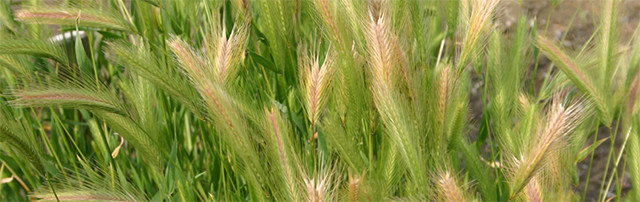# Cost for resource handling and shipment

This price list is valid from October 1, 2019

Base of calcuration No. Unit cost (yen) Cost for Okayama Univ Cost for card payment Total (yen)
1) Handling a 1,400 A0 = a × 1,400 B0 = 1,375 + (b × 0.055) A0 + B0
2) Preparation (1) Accession per line c 520 A1 = c × 520 × h B1 = A1 × 0.253 × h A1 + B1
(2) Material set (e.g. population) per line d 210 A2 = d × 210 × h B2 = A2 × 0.253 × h A2 + B2
(3) DNA clone per clone e 2,000 A3 = e × 2,000 × h B3 = A3 × 0.253× h A3 + B3
(4) DNA filter per filter f 22,000 A4 = f × 22,000 × h B4 = A4 × 0.253 × h A4 + B4
(5) Plate DNA per plate g 3,700 A5 = g × 3,700 × h B5 = A5 × 0.253 × h A5 + B5
3) Total AA = A0 + A1 + A2 + A3 + A4 + A5 BB = B0 + B1 + B2 + B3 + B4 + B5 AA + BB
(Total payment)

Cost (yen)
Postage (up to 300g) Japan b = 520
Asia b = 1,400
N America, Oceania, M East b = 2,000
Europe b = 2,200
Africa, S America b = 2,400

Rate